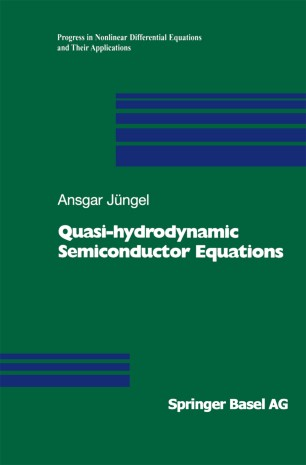# Quasi-hydrodynamic Semiconductor Equations

• Ansgar JüngelBook

Part of the Progress in Nonlinear Differential Equations and Their Applications book series (PNLDE, volume 41)

1. Front Matter
Pages i-x
2. Ansgar Jüngel
Pages 1-19
3. Ansgar Jüngel
Pages 21-26
4. Ansgar Jüngel
Pages 27-118
5. Ansgar Jüngel
Pages 119-190
6. Ansgar Jüngel
Pages 191-265
7. Back Matter
Pages 267-293

### Introduction

In this book a hierarchy of macroscopic models for semiconductor devices is presented. Three classes of models are studied in detail: isentropic drift-diffusion equations, energy-transport models, and quantum hydrodynamic equations. The derivation of each of the models is shown, including physical discussions. Furthermore, the corresponding mathematical problems are analyzed, using modern techniques for nonlinear partial differential equations. The equations are discretized employing mixed finite-element methods. Also, numerical simulations for modern semiconductor devices are performed, showing the particular features of the models.
Modern analytical techniques have been used and further developed, such as positive solution methods, local energy methods for free-boundary problems and entropy methods.
The book is aimed at applied mathematicians and physicists interested in mathematics, as well as graduate and postdoc students and researchers in these fields.

### Keywords

Finite Partial differential equations Semiconductor equation finite element method function mathematics numerical mathematics partial differential equation proof semiconductor physics

#### Authors and affiliations

• Ansgar Jüngel
• 1
1. 1.Fakultät für Mathematik und InformatikUniversität KonstanzKonstanzGermany

### Bibliographic information

• DOI https://doi.org/10.1007/978-3-0348-8334-4
• Copyright Information Birkhäuser Verlag 2001
• Publisher Name Birkhäuser, Basel
• eBook Packages
• Print ISBN 978-3-0348-9521-7
• Online ISBN 978-3-0348-8334-4
• Buy this book on publisher's site